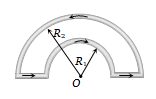• Subject:

...

• Topic:

...

The magnetic induction at the centre O in the figure shown is

(a) $\frac{{\mathrm{\mu }}_{0}\mathrm{i}}{4}\left(\frac{1}{{\mathrm{R}}_{1}}-\frac{1}{{\mathrm{R}}_{2}}\right)$                                   (b) $\frac{{\mathrm{\mu }}_{0}\mathrm{i}}{4}\left(\frac{1}{{\mathrm{R}}_{1}}+\frac{1}{{\mathrm{R}}_{2}}\right)$(c)                                       (d) $\frac{{\mathrm{\mu }}_{0}\mathrm{i}}{4}\left({\mathrm{R}}_{1}+{\mathrm{R}}_{2}\right)$